# Turnbull lectures on Colin Maclaurin, Part 2

To celebrate the bi-centenary of the death of Colin Maclaurin, Herbert Westren Turnbull, Regius Professor of Mathematics in the University of St Andrews, gave a lecture in Aberdeen on 4 February 1947. Below is the second part of his lecture which describes Maclaurin's mathematics.

For Turnbull's description of Maclaurin's life, which was the first part of his lecture, see: Turnbull on Maclaurin, Part 1

For Maclaurin's publications see: Maclaurin's publications

COLIN MACLAURIN, 1698-1746

Mathematician and Philosopher

Maclaurin made two considerable contributions to the theory of higher plane curves ; the first was his Geometria Organica sive Descriptio Linearum Curvarum Universalis (London, 1720) with the imprimatur of Sir Isaac Newton, P.R.S., 1719. The second was the Tractatus de Linearum Geometricarum Proprietatibus generalibus, which was published posthumously as an appendix to his Treatise of Algebra in 1748.

Analytical geometry had started with the epoch-making discovery of Descartes in 1637 when he solved the ancient Apollonian problem on the locus Ad tres et quattuor lineas by a systematic use of two co-ordinates $x$ and $y$ to which all the lines of a given figure could be referred. The analytical statement of this problem is tantamount to the equation $\alpha\beta = \gamma\delta$, where each of $\alpha, \beta, \gamma, \delta$ is linear in $x$ and $y$. Equated to zero each denotes a straight line.

This illustrates the title of the locus for the case when four distinct lines are involved; and the equation so found, being of the second degree in $x$ and $y$, is that of a conic. It suggested to Descartes a generalisation containing two such products of factors, each involving not merely a pair of factors $\alpha, \beta$, but $n$ factors: and in this way a curve of order $n$ was exhibited.

The process not only demonstrated the existence of such a curve but gave a geometrical law for its description. This discovery of Descartes naturally suggested a general problem, to find all possible curves of a given order $n$, and how to describe them. The case $n = 2$, that of conic sections, had been well-nigh completely worked out by the ancients, although Newton added some notable new methods of generating the curves. Newton himself considered the next case, that of cubic curves, $n = 3$, which led to a system of seventy-two varieties to which half a dozen more, that he had overlooked, were added by various later geometers, including Stirling, the friend of Maclaurin.

The general problem evidently attracted Maclaurin from the outset, and he set himself the task of finding as many types as possible of curves of order $n$, by a gradational approach through curves of lower order. As he remarks in the preface (p. 3):
Newton was the first to give the organic description of curves up to cubics. Following him we base out investigation on continuous motion of angles, whose vertices move on fixed straight lines or curves of any order less than n, when we are considering curves of order n.
He further remarks (Scholium to Part i, p. 78) on the desirability of having a general geometrical method for describing all algebraic curves, and hopes that his method will pave the way towards that goal.Maclaurin began by proving in his own way a theorem of Newton on the conic: given fixed points S and C, and the line AE, let two angles, PSQ and PCQ, of constant sizes, rotate about their vertices S and C. If Q, the intersection of two legs, describes the line AE, then P, the intersection of the other two legs, will describe a conic which passes through S and C.

Maclaurin expressed $z$ the ordinate of Q, bilinearly in terms of $x$ and $y$ the rectangular co-ordinates of P, referred to C as origin and CS as axis of $x$: and again referred to S as origin and the same axis of $x$. Cross multiplication of the two equal values of $z$ then gave him the required second degree equation of the conic in terms of $x$ and $y$.Step by step he advanced this procedure, first by allowing one angular point N to become unpivoted and made to travel along a given line, while one of its legs passes through S. As the point Q describes a straight line, the point P then traces a cubic curve with a node at C. Next he freed both angular points N and R from the fixed pivots, while requiring one leg of each to pass through them. If three of the points, N, Q, R describe straight lines, the fourth P then traces a quartic curve. Once the analytical equation of his curve had been reached he made the greatest use of purely geometrical reasoning to discover its leading properties; and here, for example, he proved the satisfying result that if any three of the points N, P, Q, R, describe straight lines then the fourth must describe a quartic. For example, when P, Q, R, describe straight lines then N describes a quartic having a triple point at C (Prop. 19, Corollary 1).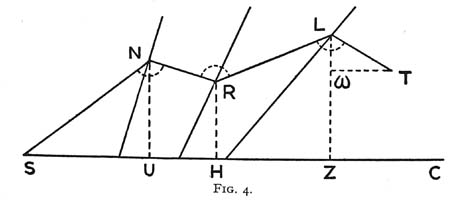To examine curves of a general order he introduced a serrated angle SNRLT, obtained by letting any number of angles of given sizes have their vertices N, R, L upon fixed guiding lines, and one free leg NS pivot on S. To evaluate Lω : Tω, the gradient of the last line of the chain, he gave a remarkable lemma, that
if any x and y occur to one dimension in the perpendicular NU upon SC, then the ratio Lω : Tω is expressible as quantities which contain x and y to one dimension, that is $px + qy + r : sx + ty + u$.
It is a clear example of the persistence of a bilinear relation for the gradient of one line, which is related to that of another line, and is a considerable foreshadowing of projective analytical geometry. Indeed Maclaurin's proof would apply equally well to the case when the gradients of each consecutive pair of broken lines NR, RL are in a (1, 1) correspondence, which is not necessarily caused by the constancy of the angle NRL. Maclaurin renders this effective by showing next that, if NU, the first ordinate along the chain of points, N, R, L involves $x$ and $y$ bilinearly as in the above gradient, then the $n$th of these ordinates is a rational fractional expression in $x$ and $y$ of order $n$.

Two such serrated angles CNRTQ and SMLKQ can be envisaged, issuing from the pivots C and S, the first with $m$ and the second with $n$ guiding lines traversed by the $m$ points N, R, T and the $n$ points M, L, K, respectively. Let their initial legs CN, SM meet in P, and the last TQ, KQ in Q. Let LK the $r$th leg from S and RT the $s$th leg from C meet in O. Maclaurin now has three variable points P, Q, O; what happens if Q describes a straight line? He finds that O describes a curve of order $(m + 1)s + (n + 1)r$, while P describes one of order $m + n + 2$.

Further extensions occur, by allowing the guiding lines upon which the angular points N, R, etc., move to be given curves, starting of course with the case of the conic. The most general result is a curve "of order $lkitpuz \times (ms + nr + s + r)$" where these single factors denote the seven curves upon which the seven points Q, N, R, T, M, L, K of the above figure are made to move.The figure, of course, commits him to the case $m = n = 3$; in general the formula would have $m + n + 1$ single factors $l ... z$, with the same final compound factor. The whole investigation is saturated with ideas that are fundamental in the treatment of higher plane curves, their nodes, cusps and higher multiple points, their freedoms and their intersections. He obtains the maximum number $(n - 1) (n - 2)/2$ of nodes on a curve of order $n$, and surmises, but does not completely prove, that an $n$-ic and an $m$-ic curve in general meet in $mn$ points. He gave, he says, a general demonstration of this theorem in a Supplement printed a few years later, but apparently never published. (Cf. Philosophical Transactions, 1735, 39, 146.)

The book continues with an elaborate discussion of pedal curves and chains of such curves - another general and systematic device which he invented originating in the fundamental property that the angle at which the radius vector strikes the arc is the same at the same moment for each curve of the chain. This is Maclaurin's inference from Euclid's theorem that the angle between a tangent and a chord through the point of contact on a circle is equal to the angle in the alternate segment! The pedal curve of a given plane curve with regard to a fixed point O is the locus of the foot of the perpendicular drawn from O to the tangent at a variable point of the curve.

It is hardly surprising that in such a wonderful extension of geometrical properties many well-known curves would emerge as particularly cases, having their due places in these hierarchies. Maclaurin makes this quite plain as the cases arise, by paying willing tribute to the discoveries of the ancients and to the more recent discoveries of Fermat, Nicol, Newton, to the spirals of Varignon, the conchoid of de la Hire, and the limaçon of Pascal. This wealth of beauty is poured out almost incidentally as the young geometer pursues his way opening up a vast new field and foreshadowing many a theorem or a geometrical principle that was to be significant in the still distant future -- the Cremona transformations, the theory of residuation of Sylvester. Maclaurin had one guiding principle to go on -- that which Newton suggested in the angular movements for the description of conics and cubics. In this Maclaurin was fortunate, but he alone of all who followed Newton showed the true significance of the device.

There is a sequel. Just before Maclaurin set out on his Continental tour he learnt from his old friend Professor Simson, of the rediscovery of a particular porism of Pappus on a variable triangle whose vertices move on three concurrent lines while its sides pass through three collinear points. (This property is virtually that of Desargues' Theorem on perspective triangles.)This at once set Maclaurin thinking again about angular movements, only instead of two pivots he used three or more, and for his angles he took those of 180°, that is, straight lines. 'Twas this led me to consider this subject anew', he remarked; and as a result he discovered a new group of theorems, strikingly related to the previous group, and again based on serrated angles; only, instead of each successive leg making a given angle with the previous, it is now made to pass through a fixed point. For example, if all but one vertex of a polygon describe specified curves of orders $p, q, r, ..., t$, respectively, and each side passes through its own fixed point, the free vertex describes a curve of order $2pqr ... t$ (Fig. 6). But if all the fixed points lie on a straight line, the order of the curve is reduced to $bqr ... t$. The porism of Pappus arises when $p = q = r = 1$, and the polygon is a triangle. It was this halving of the general order of the curve when the pivotal points were collinear that struck home; for Maclaurin already knew that P would describe a conic, however many pivots there were provided that the guiding lines were straight. He worked at this in July, 1722, while at sea en route for London and Cambrai, and incidentally produced a version of Pascal's theorem, whereby he described a conic having given five of its points.

Five years later this same case of pivots and linear guides was discussed by Braikenridge, a talented geometer who taught mathematics in Edinburgh privately for some years, and who showed to Maclaurin his results: 'which, at the same time, I showed him in my papers', Maclaurin adds. Both men ultimately published their work in the Philosophical Transactions of 1735, Vol. 39, no. 436 and no. 439; they were short and beautiful accounts, which shine together like a double star in the geometrical firmament that stretches over two millennia from the porisms of Euclid to the Ausdehnungslehre of Grassmann.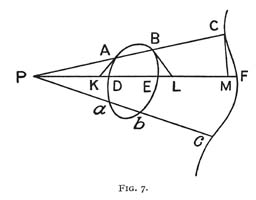A second considerable geometrical work of Maclaurin is his De Linearum Geometricarum Proprietatibus generalibus which deals with the harmonic properties of conics and plane cubic curves. Through reading de la Hire he had become acquainted with the fundamental harmonic properties of ranges and pencils of lines. These assumed a new significance when the elegant theorem discovered by Cotes, concerning a general curve, was communicated to Maclaurin by Dr Robert Smith, master of Trinity College, Cambridge, 'a gentleman not less remarkable for his learning and works, than for his fidelity and regard for his friends'.

Cotes' theorem concerns a curve of order $n$ and a point P. If a variable line through P cut the curve in $n$ points A, B, C, ..., and a point Q is taken on the line such that σPA-1 = $n$/PQ then the locus of Q is a straight line. As Maclaurin meditated on these considerations, he tells us, some other general theorems offered themselves. Whereupon 'I proceed to explain the less obvious properties of geometrical lines almost in the same order in which they occurred to me'; and what follows is a lemma that proves to be the foundation stone for the lovely edifice which he constructs. The lemma shows what can happen when two distinct ideas are perfectly blended. One came from Newton and the other from Cotes. From Newton, Maclaurin had learnt that when two straight lines PA, P$a$ meet a curve of order $n$ in the points A, B, C ..., and $a, b, c$ ... respectively, then the ratio
(PA. PB. PC ...)/(P$a$. P$b$ . P$c$ ...)

is constant for all positions of P, provided that the directions of the two lines remain fixed. Here is a property of the product of segments PA upon a variable straight line, whereas the result of Cotes concerns the sum of the reciprocals of such segments. This contrast between two expressions involving the same $n$ segments of a line fired his imagination, and suggested to Maclaurin the use of fluxions as their connecting link, or, as we should put it, logarithmic differentiation. He drew an axis PE, and allowed the point P to move along PE, while each of the lines PA and Pa moved parallel to itself. In the notation of fluxions Newton's theorem thereupon yielded the relation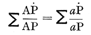(1)

where the dot can be interpreted as indicating the rate of change of the length affected. But, where AK is the tangent at A to the curve and PK is the subtangent. (This formula is nothing else than that of, for the subtangent, when E is the origin, and EP = $x$, PA = $y$ are the coordinates of A referred to oblique axes, namely EP and a line through E parallel to PA.) Hence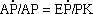, and on taking the sum of $n$ such results
σPK-1 = σPk-1

in place of (1), where P$k$ is the subtangent of the point $a$, and a common factorhas been cancelled throughout. Now if a new line P$a$ through P is taken, the left-hand side of this equality is unaltered: and vice versa if a new PA is taken. Hence this is true for any two chords through P, which cut the curve in $n$ points, so that σPK-1 is constant for all such chords. In particular if the chord closes in and ultimately coincides with PD then the points K, A, D ultimately coalesce. Thus finally
σPK-1 = σPD-1          (2)

and this result is true of any two positions of the lines PA, PD drawn through P. This is Maclaurin's Master Theorem, and this is his way of proving it. It is curious and enlightening to notice that he makes no appeal to Cotes' Theorem in the course of his proof, the two results being independent. Yet Maclaurin freely acknowledges the indirect help he received from Cotes, and in the subsequent development of the consequences Maclaurin naturally exhibits Cotes' theorem.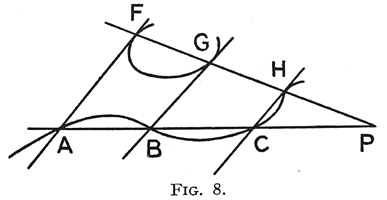I shall only briefly hint at the wonderful stream of results upon the cubic curve which follow. For example, if any chord meets the cubic in F, G, H and the tangents at F, G, H meet the curve again at A, B, C then ABC is a straight line. This is proved from the Master Theorem by taking P to be the point common to the lines AB and FGH, and applying the formula (2) to those points where the tangents at F, G, H meet PA and where the curve meets PA, so that, let us say,
1/PA + 1/PB + 1/PM = 1/PA + 1/PB + 1/PN.

This means that M, the point where AB meets the curve again, is the same as N, where the tangent at H meets the curve: and it implies that M, N, C are the same point.All possible cases, of coalescing points and failures to reach real points of intersection, are considered. Particular attention is given to the points of inflexion; for instance, if more than one inflexion exists there must be three at least, and they lie by threes upon straight lines. Let A, B, C coalesce in the above figure, at an inflexion; then the three tangents from the inflexion touch the curve at three collinear points. From any ordinary point A on the curve exactly four tangents can be drawn; and if F, G, $f, g$ are their points of contact any pair of opposite sides of this quadrilateral FG$fg$ meet at a point on the curve and also divide harmonically any chord through A. This last result, in its simpler form, when A is an inflexion on the curve, is so beautiful that it must be quoted in full. If ABC is any chord through the inflexion A, and the tangents from A touch the curve at the collinear points FGH, let the Master Theorem be applied to the lines ABC and FGH. Then
1/PA + 1/PB - 1/PC = 3/PA

and immediately it follows that A, B, P, C form a harmonic range, 'a property of the cubic of admirable simplicity'.

That Maclaurin was not only a geometer his Treatise of Fluxions abundantly shows. The book was written as a reply to a philosophical attack upon Newton's method of fluxions which Dr Berkeley, bishop of Cloyne, had made in a treatise entitled The Analyst (1734). Maclaurin was moved to vindicate his favourite study by answering the objections thus raised. But as he proceeded, so many discoveries and so many new theorems and problems occurred to him that instead of a short pamphlet he wrote a considerable work of two volumes which takes its place as one among the supreme works on mathematical analysis. It was written in English and published at Edinburgh in 1742, and contained a mature and systematic account of Newton's fluxions, set out both in geometrical and in analytical form, with a wealth of applications and many novel discoveries. It was the first logical and systematic account of fluxions, and in point of rigour could hold its own with the geometrical method of exhaustions of the Greeks and the subsequent work of Cauchy and of Weierstrass. One of the great passages in the book concerns the attraction of an ellipsoid, where Maclaurin successfully extended the work of Newton upon spherical attraction, and incidentally opened a new field of geometrical investigation by studying confocal conics. So powerfully did his synthetic methods work that Clairaut after reading the book abandoned analysis and attacked the problem of the figure of the Earth by pure geometry: and later again Lagrange described this passage of the book as 'le chef d'oeuvre de géometrie qu'on peut comparer à tout ce qu'Archimèdes nous a laissé de plus beau et de plus ingenieux' (Mém. de l'Acad. de Berlin, 1773). This was the year in which the next big step forward was taken in the theory, when Lagrange introduced the idea of potential.

Maclaurin also showed that a spheroid was a possible form of equilibrium of a mass of homogeneous liquid rotating about an axis passing through its centre of mass. The book includes a discussion on the tides: this part had been previously published, in 1740, and had received a prize from the French Academy. The purely analytical part of the book is distinguished by the same inclusiveness as characterised his earliest work, the Organica. He lays down a general principle, develops it, and shows, almost casually but explicitly, how this and that particular result or formula, of Bernoulli, De Moivre, Brook Taylor or Stirling, emerges as part of a sustained theory. He gives full credit to his forerunners, as for instance when he describes his well-known expansion (II, §6 751, p. 610)
$f(x) = f(0) + xf'(0) + x^{2}/2! f"(0) + ...$

which he presented in the form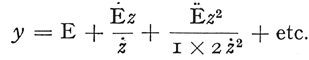as a special case of the series for $f(x + h)$ given by Brook Taylor in 1715. An earlier passage contains the well-known integral test for the convergence or divergence of a monotonic series, whereand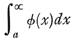are compared; a method which Cauchy rediscovered many years later. This Maclaurin turned to practical account by developing a technique for computing the series from the integral, or vice versa, to a high order of accuracy, and gave him what is nowadays known as the Euler-Maclaurin summation formula, both mathematicians having discovered the method, as it would seem, independently and nearly contemporaneously.

Perhaps the finest part of the whole work is that upon the Lines of Swiftest Descent and the isoperimetrical problems. Here Maclaurin expounds the calculus of variations by his geometrical and fluxional method, after first alluding to the early discoveries of Newton and James Bernoulli, on the solid of least resistance and the line of swiftest descent. For a particle falling under gravity, such a path, the brachistochrone, is a cycloid. It is this result of Bernoulli's which is here given.
'In pursuit of our principal design in this treatise', says Maclaurin, 'we shall endeavour to illustrate this subject which is commonly considered as one of its most abstruse parts, by proposing the resolution and composition of these problems ... in such a manner as may suggest a synthetic demonstration that may serve to verify the solution.'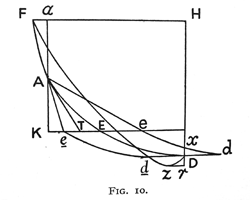The problem is to find the line of swiftest descent from F to D: but instead of doing this Maclaurin finds such a line from F to a vertical line HD through D, and then shows that this also solves his problem.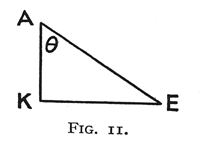A lemma shows that if a particle describe a line AE with velocity $u$, starting from A, and another particle describe KE with a greater velocity $a$, starting from K, where AKE is a right angle, then the difference of their times (which he writes T. AE and T. KE) is a minimum when $\sin \theta = u/a$. A very beautiful argument then shows that the same is true when the path AE is a finite arc of the curve FAED, the velocity at all points being that due to gravity from the height FH, while $a$ is taken as the velocity attained at D, and T . KE is, as before, the time taken to describe KE with velocity $a$.

This argument depends on two steps: first he shows that the desired curve FD cannot drop below the level of D, otherwise, if it reach $z$, the time along a horizontal path $zr$, would be less than that along $z$D, else it would describe a shorter path at a greater speed, and so shorten the time from F to the vertical H$r$. Next he takes any point e or $e$, other than E on KE, and draws the arcs ed or $ed$ through them, identical with the arc ED, and readily completes his argument by using the equality of the times in ed, ED, $ed$.

Thereupon he allows E to approach A and obtains, from minimalising T. AE - T. KE, the relation, where $x$ = F$a$, and $s$ = arc FA. This characterises a cycloid, for the property will be true of any such point A on the curve. But he does not rest satisfied until he has also given the synthetic demonstration that the curve which has this property is the line of swiftest descent. This is done by taking three points A, E, B on such a curve and their tangents AT, T$t$, $t$B, and considering the time in the path AT, T$t$, $t$B due to velocities under gravity at A, E and B respectively, as also the time in $ab$ (A$a$, B$b$ being ordinates from the horizontal F$b$) for a constant velocity (that at D) as before.

He proves T . AT + T . T$t$ + T . $t$B - T . ab to be minimal compared with that of any other polygon from A to the points T, $t$, B taken elsewhere upon their horizontal levels. This time is further reduced by inserting further tangents, touching the curve at the levels T and t; and repeating the argument. A limit is reached with the time on the actual curve, diminished as always by T. ab. But this last is constant throughout the process, so that the time on the arc AEB itself is a minimum.The same care is shown in all the problems of this nature with which the book abounds. It is also clear that in his treatment of the velocities due to a gravitational field stratified into levels, as in the above example, or again into concentric circles as in what followed for the case of gravitation towards a fixed centre, Maclaurin came near to foreshadowing Lagrange's concept of the potential.

Here we must take leave of the mathematics so well adorned by the genius of Maclaurin's thought. He continued the great work which Newton had begun, and to-day we are still indebted to him for the discoveries and the processes with which he has enriched our mathematical heritage.

For Turnbull's description and assessment of Maclaurin's life, which was the first part of his lecture see: Turnbull on Maclaurin, Part 1

For Maclaurin's publications see: Maclaurin's publications

Last Updated April 2007